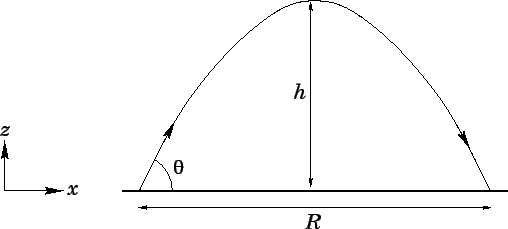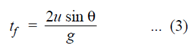# Horizontal Projectile Motion: Physics Notes

When an object is thrown from horizontal at an angle θ except 90°, then it will follow a trajectory and the motion is called projectile motion.  A horizontally thrown ball and a bullet fired from a rifle held horizontally are the examples of projectiles in the horizontal direction. For this type of projection there is an initial velocity u only in the horizontal or x-direction. But there is no initial velocity in the vertical or y-direction.

However, there is acceleration in the downward direction due to gravity. Since there is no acceleration or force in the x-direction after it is projected, the projectile moves in this direction with a constant speed (u).

As the object moves horizontally, it also falls in the downward direction due to gravity. In the downward direction, the motion is the same as that of a dropped object.

Let us consider a body A which is allowed to fall freely and another body B projected horizontally with a velocity u from the same height and at the same time. The body B possesses simultaneously:

• Uniform horizontal velocity u
• A non-uniform vertical velocity v.

As the body B travels down its vertical velocity (v) increases due to acceleration due to gravity. But the horizontal velocity u remains constant. Hence the body A which is freely falling and the body B projected horizontally from the same height at the same time will strike the ground simultaneously at different points. But the two bodies at any instant will be at the same vertical height above the ground. Thus the motion of a freely falling body is same as that of a horizontally thrown projectile. A stone released from a moving train behaves like the horizontal projectile B. As the path of B is a parabola, a stone released from a moving train also follows a parabolic path.

##### Oblique projection Motion

Consider a body which is projected at an angle with the horizontal. Let u be the initial velocity of the projectile and θ be the angle of projection.Initial velocity can be resolved into two components viz. (i) the horizontal component u cos θ and (ii) the vertical component u sin θ. The path of the projectile ACB is a parabola and CD (h) is the maximum height reached by it.  The time (t) taken by the projectile to reach the maximum height is given byThe maximum height reached is given byThe time of flight (tf) of a projectile is defined as the time taken by it to reach the horizontal plane after its projection. It s given byThe distance between the point of projection A and the point B where the projectile strikes the horizontal plane again is called its range (R). It is given byEquation (4) shows that the range is maximum when θ = 45° (because sin 90°= 1). This is a consideration in several sports

Events such as shot-put, javelin and golf where maximum ranges are desired. The player is expected to throw at an angle of 45° to achieve maximum range.

•##### M BISHT

Plz sir.

•##### Master Chie

Correct the spelling in

“Velocity of a body dropped from a hight”.

•##### Master Chief
•